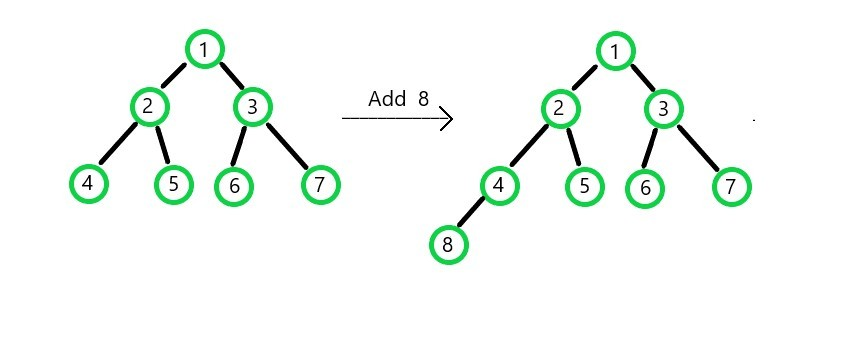# Complexity analysis of various operations of Binary Min Heap

A Min Heap is a Complete Binary Tree in which the children nodes have a higher value (lesser priority) than the parent nodes, i.e., any path from the root to the leaf nodes, has an ascending order of elements. In the case of a binary tree, the root is considered to be at height 0, its children nodes are considered to be at height 1, and so on. Each node can have two children at max.

Important properties for Min Heap:
1. Parent node will always have higher priority and lesser value than the child node (in case of Min Heaps).
2. Heap is a complete binary tree. So in order to fill the Nth level, (N-1) levels should be completely filled first and the filling of nodes in the Nth level should take place from left to right.

Based on these properties various operations of Min Heap are as follow:

1. Complexity Analysis of Insert operation in Min Heap
When a node is supposed to add into the heap, the element is added at the next vacant index of the array. Then it is checked whether the inserted child node is in accordance with the parent node or not. If the child has a lower value (higher priority) than the parent, the swapping of the nodes is done. This swapping process goes on until the properties of Min Heap are fulfilled.If a node is to be inserted at a level of height H:

Complexity of adding a node is: O(1)

Complexity of swapping the nodes(upheapify): O(H)
(swapping will be done H times in the worst case scenario)

Total complexity: O(1) + O(H) = O(H)

For a Complete Binary tree, its height H = O(log N), where N represents total no. of nodes.

Therefore, Overall Complexity of insert operation is O(log N).

2. Complexity Analysis of Delete operation in min heap

Deletion of a node cannot be done randomly. The element with the highest priority (i.e. parent) will be deleted first followed by the next node in order of priority. This is why heap is called a priority queue.
First, swap the positions of the parent node and leaf node, and then remove the newly formed leaf node (which was originally the parent) from the queue. Next, start the swapping process so that the new parent node is placed in the right position in accordance with the properties of Min Heap.

If a node is to be deleted from a heap with height H:

Complexity of swapping parent node and leaf node is: O(1)

Complexity of swapping the nodes(downheapify): O(H)
(swapping will be done H times in the worst case scenario)

Total complexity: O(1) + O(H) = O(H)

For a Complete Binary tree, its height H = O(log N), where N represents total no. of nodes.

Therefore, Overall Complexity of delete operation is O(log N).

3. Complexity of getting the Minimum value from min heap

In order to obtain the minimum value just return the value of the root node (which is the smallest element in Min Heap), So simply return the element at index 0 of the array.

Hence, Complexity of getting minimum value is: O(1)

Attention reader! Don’t stop learning now. Get hold of all the important DSA concepts with the DSA Self Paced Course at a student-friendly price and become industry ready.

My Personal Notes arrow_drop_upIf you like GeeksforGeeks and would like to contribute, you can also write an article using contribute.geeksforgeeks.org or mail your article to contribute@geeksforgeeks.org. See your article appearing on the GeeksforGeeks main page and help other Geeks.

Please Improve this article if you find anything incorrect by clicking on the "Improve Article" button below.

Article Tags :
Practice Tags :

Be the First to upvote.

Please write to us at contribute@geeksforgeeks.org to report any issue with the above content.